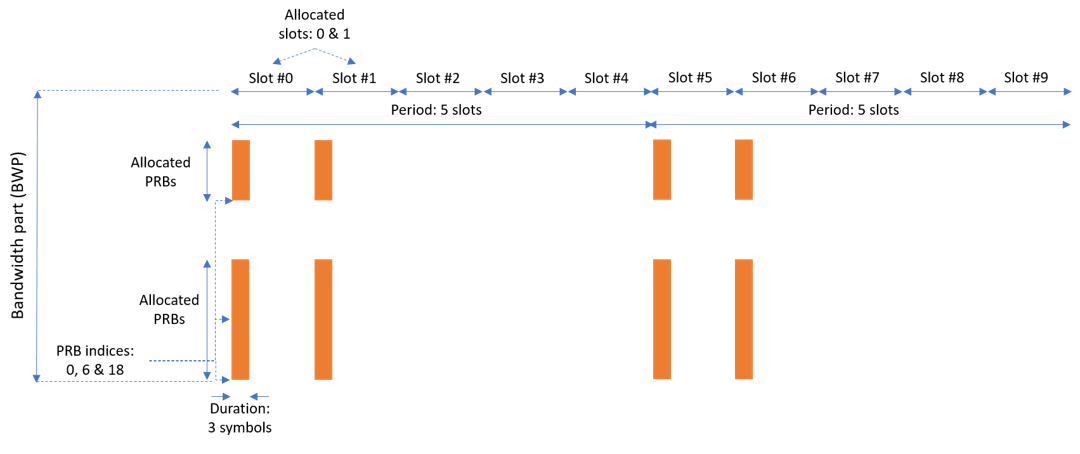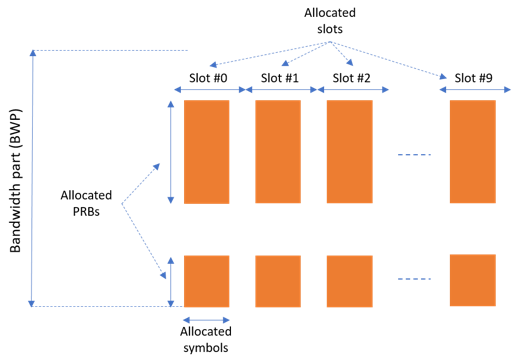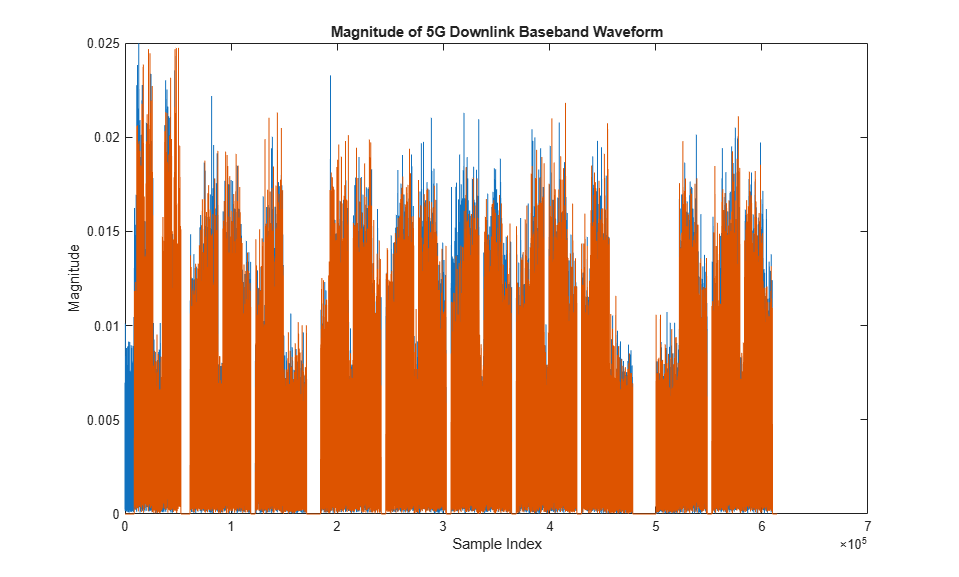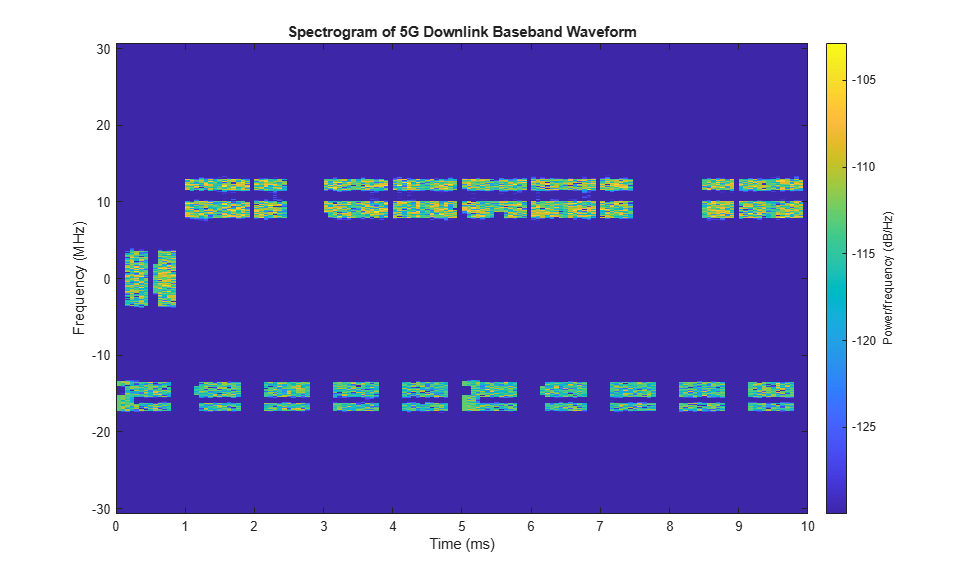# 5G NR Downlink Carrier Waveform Generation

This example shows how to use the 5G NR downlink carrier waveform generator to create a baseband component carrier waveform.

### Introduction

This example shows how to parameterize and generate a 5G New Radio (NR) downlink waveform using `nrWaveformGenerator`. The following channels and signals can be generated:

• PDSCH and its associated DM-RS and PT-RS

• PDCCH and its associated DM-RS

• PBCH and its associated DM-RS

• PSS and SSS

• CSI-RS

This example supports the parameterization and generation of multiple SCS specific carriers and multiple bandwidth parts (BWP). Multiple instances of the PDSCH and PDCCH channels can be generated over the different BWPs. Sets of CORESETs and search space monitoring opportunities can be configured for mapping the PDCCHs. Note that no precoding is applied to the physical channels and signals in this example.

### Waveform and Carrier Configuration

The baseband waveform is parameterized by an `nrDLCarrierConfig` object and a set of additional objects associated with its channels and signals. This section sets the SCS specific carrier bandwidths in resource blocks, the cell ID, and the length of the generated waveform in subframes. You can control SCS carrier bandwidths and guardbands using the `NStartGrid` and `NSizeGrid` parameters.

```waveconfig = nrDLCarrierConfig(); % Create an instance of the waveform's parameter object waveconfig.NCellID = 0; % Cell identity waveconfig.ChannelBandwidth = 40; % Channel bandwidth (MHz) waveconfig.FrequencyRange = 'FR1'; % 'FR1' or 'FR2' waveconfig.NumSubframes = 10; % Number of 1ms subframes in generated waveform (1,2,4,8 slots per 1ms subframe, depending on SCS) % Define a set of SCS specific carriers, using the maximum sizes for a % 40 MHz NR channel. See TS 38.101-1 for more information on defined % bandwidths and guardband requirements scscarriers = {nrSCSCarrierConfig(),nrSCSCarrierConfig()}; scscarriers{1}.SubcarrierSpacing = 15; scscarriers{1}.NSizeGrid = 216; scscarriers{1}.NStartGrid = 0; scscarriers{2}.SubcarrierSpacing = 30; scscarriers{2}.NSizeGrid = 106; scscarriers{2}.NStartGrid = 1; ```

### SS Burst

In this section you can set the parameters for the SS burst. The numerology of the SS burst can be different from other parts of the waveform. This is specified via the block pattern parameter as specified in TS 38.213 Section 4.1. A bitmap is used to specify which blocks are transmitted in a 5ms half-frame burst. The periodicity in milliseconds and the power of the burst can also be set here. Other SS burst parameters not shown here can also be set. For the full list see the help for `nrWavegenSSBurstConfig`.

```% SS burst configuration ssburst = nrWavegenSSBurstConfig(); ssburst.Enable = 1; % Enable SS Burst ssburst.Power = 0; % Power scaling in dB ssburst.BlockPattern = 'Case B'; % Case B (30kHz) subcarrier spacing ssburst.TransmittedBlocks = [1 1 1 1]; % Bitmap indicating blocks transmitted in a 5ms half-frame burst ssburst.Period = 20; % SS burst set periodicity in ms (5, 10, 20, 40, 80, 160) ssburst.NCRBSSB = []; % Frequency offset of SS burst (CRB), use [] for the waveform center ```

### Bandwidth Parts

A BWP is formed by a set of contiguous resources sharing a numerology on a given carrier. This example supports the use of multiple BWPs using a cell array. Each entry in the array represents a BWP. For each BWP you can specify the subcarrier spacing (SCS), the cyclic prefix (CP) length and the bandwidth. The `SubcarrierSpacing` parameter maps the BWP to one of the SCS specific carriers defined earlier. The `NStartBWP` parameter controls the location of the BWP in the carrier, relative to point A. This is expressed in terms of the BWP numerology. Different BWPs can overlap with each other.```% Bandwidth parts configurations bwp = {nrWavegenBWPConfig(),nrWavegenBWPConfig()}; bwp{1}.BandwidthPartID = 1; % Bandwidth part ID bwp{1}.SubcarrierSpacing = 15; % BWP subcarrier spacing bwp{1}.CyclicPrefix = 'Normal'; % BWP cyclic prefix for 15 kHz bwp{1}.NSizeBWP = 25; % Size of BWP bwp{1}.NStartBWP = 12; % Position of BWP, relative to point A (i.e. CRB) bwp{2}.BandwidthPartID = 2; % Bandwidth part ID bwp{2}.SubcarrierSpacing = 30; % BWP subcarrier spacing bwp{2}.CyclicPrefix = 'Normal'; % BWP cyclic prefix for 30 kHz bwp{2}.NSizeBWP = 50; % Size of BWP bwp{2}.NStartBWP = 51; % Position of BWP, relative to point A (i.e. CRB) ```

### CORESET and Search Space Configuration

The parameters in this section specify the control resource set (CORESET) and the PDCCH search space configuration. The CORESET and search spaces specify the possible locations (in time and frequency) of the control channel transmissions for a given numerology. The generator supports multiple CORESETs and search spaces. The following parameters can be specified:

• The OFDM symbols which specify the first symbol of each CORESET monitoring opportunity in a slot

• The duration of the block of allocated slots within a period

• Periodicity of the allocation pattern

• The CORESET duration in symbols, either 1, 2 or 3

• A bitmap defining the allocated physical resource blocks (PRB) of the CORESET. Note that the CORESET frequency allocation is defined in blocks of 6 PRBs, aligned in common resource block (CRB) numbering, i.e. relative to point A. Each bit in the bitmap selects all 6 PRBs in the CRB aligned block that contains it.

• CCE-to-REG mapping which can be 'interleaved' or 'noninterleaved'

• Resource-element group (REG) bundle size (L), either (2,6) or (3,6), based on CORESET duration

• Interleaver size, either 2, 3, or 6

• Shift index, a scalar value in range 0...274

The figure below shows the meaning of some of the CORESET parameters.```% CORESET and search space configurations coresets = {nrCORESETConfig()}; coresets{1}.CORESETID = 1; % CORESET ID coresets{1}.Duration = 3; % CORESET symbol duration (1,2,3) coresets{1}.FrequencyResources = [1 1 0 1]; % Bitmap indicating blocks of 6 PRB for CORESET (RRC - frequencyDomainResources) coresets{1}.CCEREGMapping = 'noninterleaved'; % Mapping: 'interleaved' or 'noninterleaved' coresets{1}.REGBundleSize = 3; % L (2,6) or (3,6) coresets{1}.InterleaverSize = 2; % R (2,3,6) coresets{1}.ShiftIndex = waveconfig.NCellID; % Set to NCellID searchspaces = {nrSearchSpaceConfig()}; searchspaces{1}.SearchSpaceID = 1; % Search space ID searchspaces{1}.CORESETID = 1; % CORESET associated with this search space searchspaces{1}.SearchSpaceType = 'ue'; % Search space type, 'ue' or 'common' searchspaces{1}.SlotPeriodAndOffset = [5,0]; % Allocated slot period and slot offset of search space pattern searchspaces{1}.Duration = 2; % Number of slots in the block of slots in pattern period searchspaces{1}.StartSymbolWithinSlot = 0; % First symbol of each CORESET monitoring opportunity in a slot searchspaces{1}.NumCandidates = [8 8 4 2 0]; % Number of candidates at each AL (set to 0 if the AL doesn't fit in CORESET) ```

### PDCCH Instances Configuration

This section specifies the parameters for the set of PDCCH instances in the waveform. Each element in the structure array defines a PDCCH sequence instance. The following parameters can be set:

• Enable/disable the PDCCH sequence

• Specify the BWP carrying the PDCCH

• PDCCH instance power in dB

• Enable/disable DCI channel coding

• Allocated search spaces within the CORESET monitoring occasion sequence

• Search space (and CORESET) which carries the PDCCH instances

• Periodicity of the allocation. If this is set to empty it indicates no repetition

• The aggregation level (AL) of the PDCCH (number of control channel elements (CCEs))

• The allocated candidate which specifies the CCE used for the transmission of the PDCCH

• RNTI

• Scrambling NID for this PDCCH and its associated DM-RS

• DM-RS power boosting

• DCI message data source. You can use an array of bits or one of the following standard PN sequences: 'PN9-ITU', 'PN9', 'PN11', 'PN15', 'PN23'. The seed for the generator can be specified using a cell array in the form `{'PN9',seed}`. If no seed is specified, the generator is initialized with all ones

```pdcch = {nrWavegenPDCCHConfig()}; pdcch{1}.Enable = 1 ; % Enable PDCCH sequence pdcch{1}.Power = 1.1; % Power scaling in dB pdcch{1}.Coding = 1; % Enable DCI coding pdcch{1}.BandwidthPartID = 1; % Bandwidth part pdcch{1}.SearchSpaceID = 1; % Search space pdcch{1}.SlotAllocation = 0; % Slots used with period pdcch{1}.Period = 5; % Period of transmission pattern pdcch{1}.AggregationLevel = 8; % Aggregation level (1,2,4,8,16 CCEs) pdcch{1}.AllocatedCandidate = 1; % PDCCH candidate in search space (1 based) pdcch{1}.RNTI = 0; % RNTI pdcch{1}.DMRSScramblingID = 1; % PDCCH and DM-RS scrambling NID pdcch{1}.DMRSPower = 0; % Additional power boosting in dB pdcch{1}.DataBlockSize = 20; % DCI payload size pdcch{1}.DataSource = 'PN9'; % DCI data source ```

### PDSCH Instances Configuration

This section specifies the set of PDSCH instances in the waveform. Each element in the cell array of `nrWavegenPDSCHConfig` defines a PDSCH sequence instance. This example defines two PDSCH sequence instances.

General Parameters

Set these parameters for each PDSCH sequence instance:

• Enable or disable this PDSCH sequence

• Specify the BWP carrying the PDSCH. The PDSCH will use the SCS specified for this BWP

• Power scaling in dB

• Enable or disable DL-SCH transport channel coding

• Transport block data source. You can use an array of bits or one of the following standard PN sequences: 'PN9-ITU', 'PN9', 'PN11', 'PN15', 'PN23'. The seed for the generator can be specified using a cell array in the form `{'PN9', seed}`. If no seed is specified, the generator is initialized with all ones

• Target code rate used to calculate the transport block sizes

• Symbol modulation

• Number of layers

• Redundancy version (RV) sequence

• Enable or disable the interleaving of the virtual to physical resource block mapping. If this parameter is not specified, the direct, non-interleaved mapping is considered

• Bundle size for the interleaved map, specified by the higher layer parameter vrb-ToPRB-Interleaver. If this parameter is not specified, the bundle size is set to 2

```pdsch = {nrWavegenPDSCHConfig()}; pdsch{1}.Enable = 1; % Enable PDSCH sequence pdsch{1}.BandwidthPartID = 1; % Bandwidth part of PDSCH transmission pdsch{1}.Power = 0; % Power scaling in dB pdsch{1}.Coding = 1; % Enable DL-SCH transport channel coding pdsch{1}.DataSource = 'PN9'; % Channel data source pdsch{1}.TargetCodeRate = 0.4785; % Code rate used to calculate transport block sizes pdsch{1}.XOverhead = 0; % Rate matching overhead pdsch{1}.Modulation = 'QPSK'; % 'QPSK', '16QAM', '64QAM', '256QAM' pdsch{1}.NumLayers = 2; % Number of PDSCH layers pdsch{1}.RVSequence = [0,2,3,1]; % RV sequence to be applied cyclically across the PDSCH allocation sequence pdsch{1}.VRBToPRBInterleaving = 0; % Disable interleaved resource mapping pdsch{1}.VRBBundleSize = 2; % vrb-ToPRB-Interleaver parameter ```

Allocation

The following diagram represents some of the parameters used in the PDSCH allocation.You can set the following parameters to control the PDSCH allocation. Note that these parameters are relative to the BWP. The specified PDSCH allocation will avoid the locations used for the SS burst.

• Symbols in a slot allocated to each PDSCH instance

• Slots in a frame used for the sequence of PDSCH

• Period of the allocation in slots. If this is empty it indicates no repetition

• The allocated PRBs are relative to the BWP

• RNTI. This value is used to link the PDSCH to an instance of the PDCCH

• NID for scrambling the PDSCH bits

```pdsch{1}.SymbolAllocation = [2,9]; % First symbol and length pdsch{1}.SlotAllocation = 0:9; % Allocated slot indices for PDSCH sequence pdsch{1}.Period = 15; % Allocation period in slots pdsch{1}.PRBSet = [0:5, 10:20]; % PRB allocation pdsch{1}.RNTI = 0; % RNTI pdsch{1}.NID = 1; % Scrambling for data part ```

CORESETs and sets of PRB can be specified for rate matching around, if required

• The PDSCH can be rate matched around one or more CORESETs

• The PDSCH can be rate matched around other resource allocations

```pdsch{1}.ReservedCORESET = 1; % Rate matching pattern, defined by CORESET IDs pdsch{1}.ReservedPRB{1}.PRBSet = []; % Rate matching pattern, defined by set of PRB (RRC 'bitmaps') pdsch{1}.ReservedPRB{1}.SymbolSet = []; pdsch{1}.ReservedPRB{1}.Period = []; ```

PDSCH DM-RS Configuration

Set the DM-RS parameters

```% Antenna port and DM-RS configuration (TS 38.211 section 7.4.1.1) pdsch{1}.MappingType = 'A'; % PDSCH mapping type ('A'(slot-wise),'B'(non slot-wise)) pdsch{1}.DMRSPower = 0; % Additional power boosting in dB pdsch{1}.DMRS.DMRSPortSet = [] ; % DM-RS antenna ports used ([] gives port numbers 0:NumLayers-1) pdsch{1}.DMRS.DMRSTypeAPosition = 2; % Mapping type A only. First DM-RS symbol position (2,3) pdsch{1}.DMRS.DMRSLength = 1; % Number of front-loaded DM-RS symbols (1(single symbol),2(double symbol)) pdsch{1}.DMRS.DMRSAdditionalPosition = 0; % Additional DM-RS symbol positions (max range 0...3) pdsch{1}.DMRS.DMRSConfigurationType = 2; % DM-RS configuration type (1,2) pdsch{1}.DMRS.NumCDMGroupsWithoutData = 1; % CDM groups without data (max range 1...3) pdsch{1}.DMRS.NIDNSCID = 1; % Scrambling identity (0...65535) pdsch{1}.DMRS.NSCID = 0; % Scrambling initialization (0,1) ```

PDSCH PT-RS Configuration

Set the PT-RS parameters

```% PT-RS configuration (TS 38.211 section 7.4.1.2) pdsch{1}.EnablePTRS = 0; % Enable or disable the PT-RS (1 or 0) pdsch{1}.PTRSPower = 0; % Additional PT-RS power boosting in dB pdsch{1}.PTRS.TimeDensity = 1; % Time density (L_PT-RS) of PT-RS (1,2,4) pdsch{1}.PTRS.FrequencyDensity = 2; % Frequency density (K_PT-RS) of PT-RS (2,4) pdsch{1}.PTRS.REOffset = '00'; % PT-RS resource element offset ('00','01','10','11') pdsch{1}.PTRS.PTRSPortSet = 0; % PT-RS antenna ports must be a subset of DM-RS ports % When PT-RS is enabled, the DM-RS ports must be in range 0 to 3 for DM-RS % configuration type 1 and in range 0 to 5 for DM-RS configuration type 2. % Nominally the antenna port of PT-RS is the lowest DM-RS port number. ```

Specifying Multiple PDSCH Instances

A second PDSCH sequence instance is specified next using the second BWP.

```pdsch{2} = pdsch{1}; pdsch{2}.Enable = 1; pdsch{2}.BandwidthPartID = 2; % PDSCH mapped to 2nd BWP pdsch{2}.SymbolAllocation = [0,12]; pdsch{2}.SlotAllocation = [2:4,6:20]; pdsch{2}.PRBSet = [25:30, 35:38]; % PRB allocation, relative to BWP ```

### CSI-RS

This section configures channel state information reference signals (CSI-RS) in the waveform. Each element in the cell array represents a set of CSI-RS resources associated with a BWP.

General Parameters

Set these parameters for a set of CSI-RS resources:

• Enable or disable this set of CSI-RS resources

• Specify the BWP carrying this set of CSI-RS resources. The CSI-RS resource(s) configuration will use the SCS specified for this BWP

• Specify the power scaling in dB. Providing a scalar defines the power scaling for a single CSI-RS resource or all configured CSI-RS resources. Providing a vector defines a separate power level for each of the CSI-RS resources.

```csirs = {nrWavegenCSIRSConfig()}; csirs{1}.Enable = 0; csirs{1}.BandwidthPartID = 1; csirs{1}.Power = 3; % in dB ```

CSI-RS configuration

You can configure the following parameters for one or more zero-power (ZP) or non-zero-power (NZP) CSI-RS resource configurations.

• Type of CSI-RS resource(s) ('nzp','zp')

• Row number corresponds to CSI-RS resource(s) as defined in TS 38.211 Table 7.4.1.5.3-1 (1...18)

• Frequency density of CSI-RS resource(s) ('one','three','dot5even','dot5odd')

• Subcarrier locations of CSI-RS resource(s) within a resource block (RB)

• Number of RBs allocated to CSI-RS resource(s) (1...275)

• Starting RB index of CSI-RS resource(s) allocation relative to the carrier resource grid (0...274)

• OFDM symbol locations of CSI-RS resource(s) within a slot

• The period and offset of slots (0-based) of CSI-RS resource(s). This parameter can be a vector or a cell array of vectors. In the latter case, each cell corresponds to an individual CSI-RS resource. In case of a vector, the same set of slots is used for all CSI-RS resources

• Scrambling identity corresponds to CSI-RS resource(s) for pseudo-random sequence generation (0...1023)

```csirs{1}.CSIRSType = {'nzp','zp'}; csirs{1}.RowNumber = [3 5]; csirs{1}.Density = {'one','one'}; csirs{1}.SubcarrierLocations = {6,4}; csirs{1}.NumRB = 25; csirs{1}.RBOffset = 12; csirs{1}.SymbolLocations = {13,9}; csirs{1}.CSIRSPeriod = {[5 0],[5 0]}; csirs{1}.NID = 5; ```

Specifying Multiple CSI-RS Instances

A set of CSI-RS resources associated with the second BWP.

```csirs{2} = nrWavegenCSIRSConfig(); csirs{2}.Enable = 0; csirs{2}.BandwidthPartID = 2; csirs{2}.Power = 3; % in dB csirs{2}.CSIRSType = {'nzp','nzp'}; csirs{2}.RowNumber = [1 1]; csirs{2}.Density = {'three','three'}; csirs{2}.SubcarrierLocations = {0,0}; csirs{2}.NumRB = 50; csirs{2}.RBOffset = 50; csirs{2}.SymbolLocations = {6,10}; csirs{2}.CSIRSPeriod = {[10,1],[10,1]}; csirs{2}.NID = 0; ```

### Waveform Generation

This section assigns all the channel and signal parameters into the main carrier configuration object `nrDLCarrierConfig`, then generates and plots the waveform.

```waveconfig.SSBurst = ssburst; waveconfig.SCSCarriers = scscarriers; waveconfig.BandwidthParts = bwp; waveconfig.CORESET = coresets; waveconfig.SearchSpaces = searchspaces; waveconfig.PDCCH = pdcch; waveconfig.PDSCH = pdsch; waveconfig.CSIRS = csirs; [waveform,info] = nrWaveformGenerator(waveconfig); % Plot the magnitude of the baseband waveform for the set of antenna ports defined figure; plot(abs(waveform)); title('Magnitude of 5G Downlink Baseband Waveform'); xlabel('Sample Index'); ylabel('Magnitude'); % Plot spectogram of waveform for first antenna port samplerate = info.ResourceGrids(1).Info.SampleRate; nfft = info.ResourceGrids(1).Info.Nfft; figure; spectrogram(waveform(:,1),ones(nfft,1),0,nfft,'centered',samplerate,'yaxis','MinThreshold',-130); title('Spectrogram of 5G Downlink Baseband Waveform'); ```The waveform generator function returns the time domain waveform and a structure `info` which contains the underlying resource element grid and a breakdown of the resources used by all the PDSCH and PDCCH instances in the waveform.

The `ResourceGrids` field is structure array which contains the following fields:

• The resource grid corresponding to each BWP

• The resource grid of the overall bandwidth containing the channels and signals in each BWP

• An info structure with information corresponding to each BWP. The contents of this info structure for the first BWP are shown below.

```disp('Modulation information associated with BWP 1:') disp(info.ResourceGrids(1).Info) ```
```Modulation information associated with BWP 1: Nfft: 4096 SampleRate: 61440000 CyclicPrefixLengths: [1x14 double] SymbolLengths: [1x14 double] Windowing: 0 SymbolPhases: [0 0 0 0 0 0 0 0 0 0 0 0 0 0] SymbolsPerSlot: 14 SlotsPerSubframe: 1 SlotsPerFrame: 10 k0: 0 ```

Note that the generated resource grid is a 3D matrix where the different planes represent the antenna ports. For the different physical channels and signals the lowest port is mapped to the first plane of the grid.